# Integral hyperbolic sine

(diff) ← Older revision | Latest revision (diff) | Newer revision → (diff)

The special function defined, for real, bywhere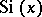is the integral sine. The integral hyperbolic sine can be represented by the series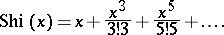It is related to the integral hyperbolic cosineby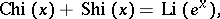where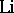is the integral logarithm.

Sometimes it is denoted by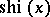.

For references see Integral cosine.

This function, which is seldom used because of its relation with the sine integral, is also called the hyperbolic sine integral. It can, of course, be defined (as above) for.